## # dom 结构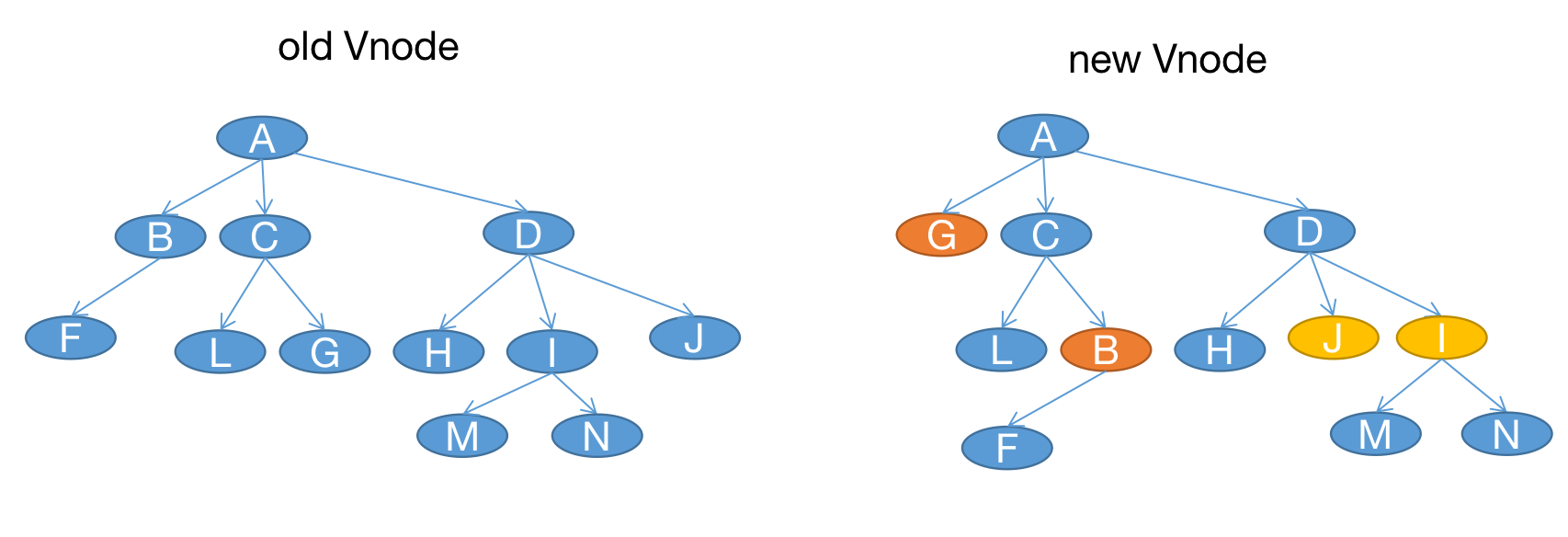for(const newVNode of newVnodeTree) {
for(const oldVNode of oldVnodeTree) {
if(isSameNode(newVNode, oldVNode)) {
newVNode.elm = oldVNodo.elm // 拿到对应的 dom
update(newVNode, oldVNode)
break;
}
}
}


update 函数需要去拿到他们各自的父节点和邻居节点，然后进行更新，时间复杂度可能是 O(n) ，再结合外层的两个 for 循环，整体的时间复杂度将是 O($n^3$)。即使 update 函数非常优秀，那么整体的时间复杂度也一定是大于 O($n^2$) 的。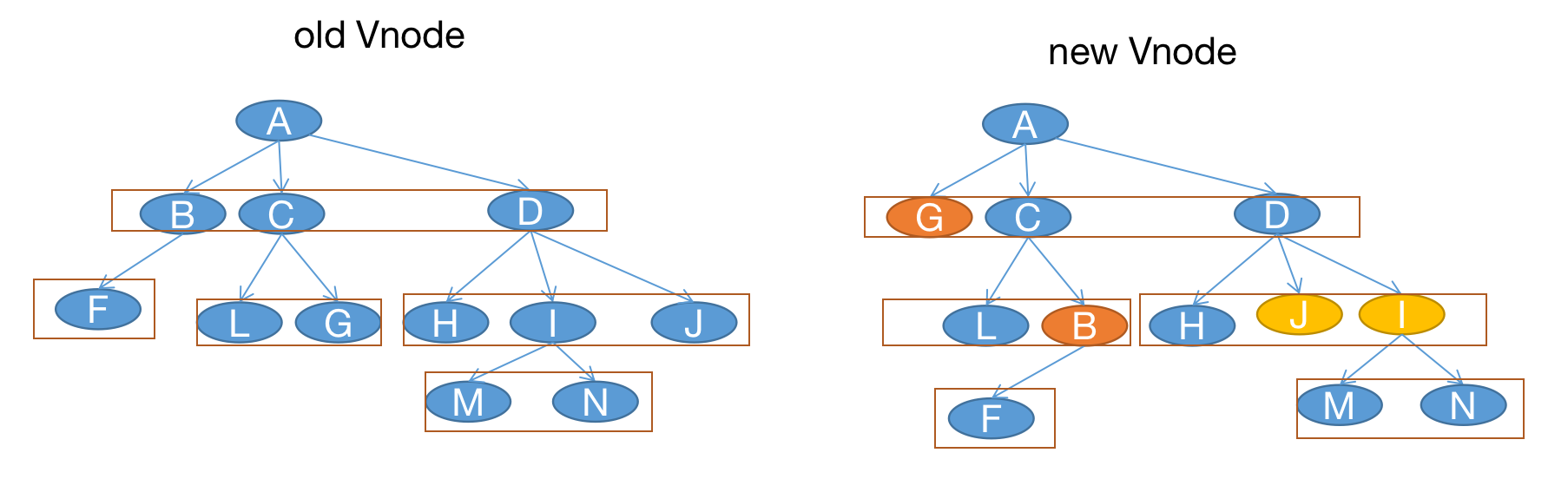for(const newVNode of newVnodeTree) {
const oldVNodo = 从当前节点的同层节点中寻找旧vnode（newVnode）
if(oldVNodo) {
newVNode.elm = oldVNodo.elm
update(newVNode, oldVNode)
}
}


## # 更新过程

function patchVnode(oldVnode, vnode) {
if (oldVnode === vnode) {
return;
}

const elm = (vnode.elm = oldVnode.elm);
const oldCh = oldVnode.children;
const ch = vnode.children;
const data = vnode.data;
if (isDef(data) && isPatchable(vnode)) {
for (i = 0; i < cbs.update.length; ++i)
cbs.update[i](oldVnode, vnode);
}
if (isUndef(vnode.text)) {
if (isDef(oldCh) && isDef(ch)) {
if (oldCh !== ch) updateChildren(elm, oldCh, ch);
} else if (isDef(oldVnode.text)) {
// 更新成了空字符
nodeOps.setTextContent(elm, "");
}
} else if (oldVnode.text !== vnode.text) {
nodeOps.setTextContent(elm, vnode.text);
}
}


function updateChildren(elm, oldCh, ch) {
for (let i = 0; i < oldCh.length; i++) {
patchVnode(oldCh[i], ch[i]);
}
}


function updateChildren(elm, oldCh, ch) {
for (let i = 0; i < ch.length; i++) {
for(let j = 0; j < oldCh.length; j++) {
if(sameVnode(ch[i], oldCh[j])) {
ch[i].elm = oldCh[j].elm;
patchVnode(oldCh[i], ch[j]);
break;
}
}
}
}


// vue 源码中的 sameVnode 判断的比较多，这里我们仅简单理解为 key、tag 一致，并且 data 属性还存在即可
function sameVnode(a, b) {
return (
a.key === b.key && a.tag === b.tag && isDef(a.data) === isDef(b.data)
);
}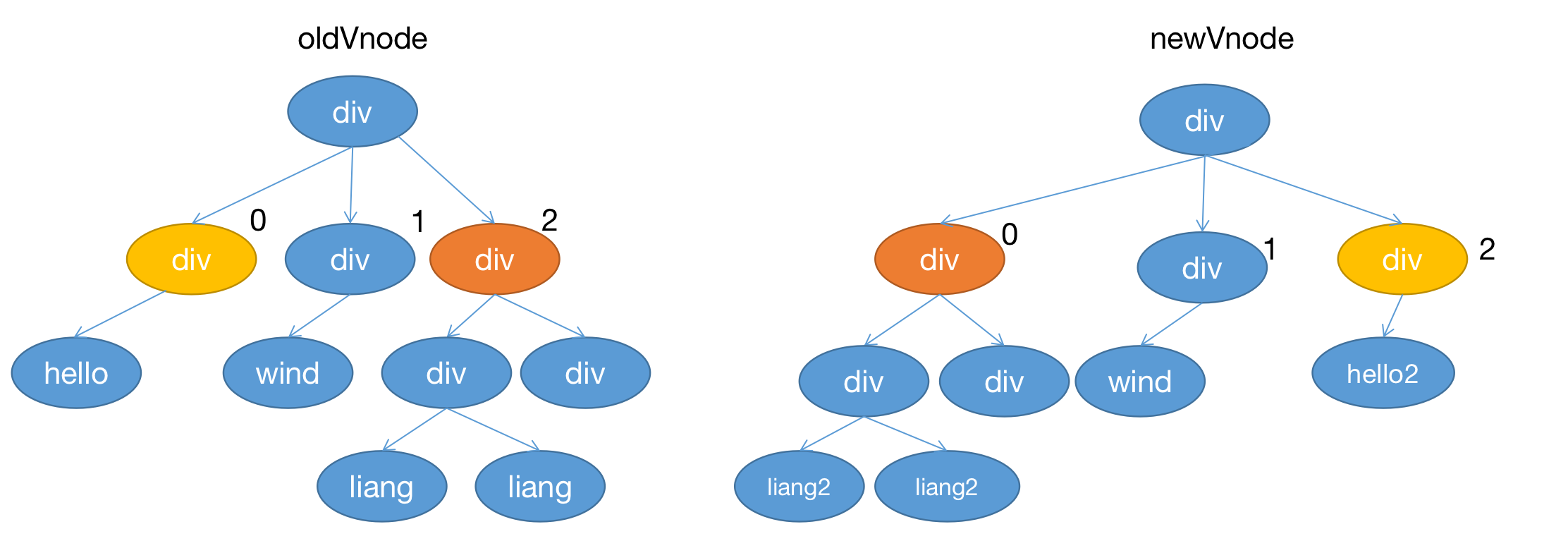function updateChildren(elm, oldCh, ch) {
for (let i = 0; i < ch.length; i++) {
for(let j = 0; j < oldCh.length; j++) {
if(sameVnode(ch[i], oldCh[j])) {
ch[i].elm = oldCh[j].elm;
patchVnode(oldCh[i], ch[j]);
break;
}
}
}
}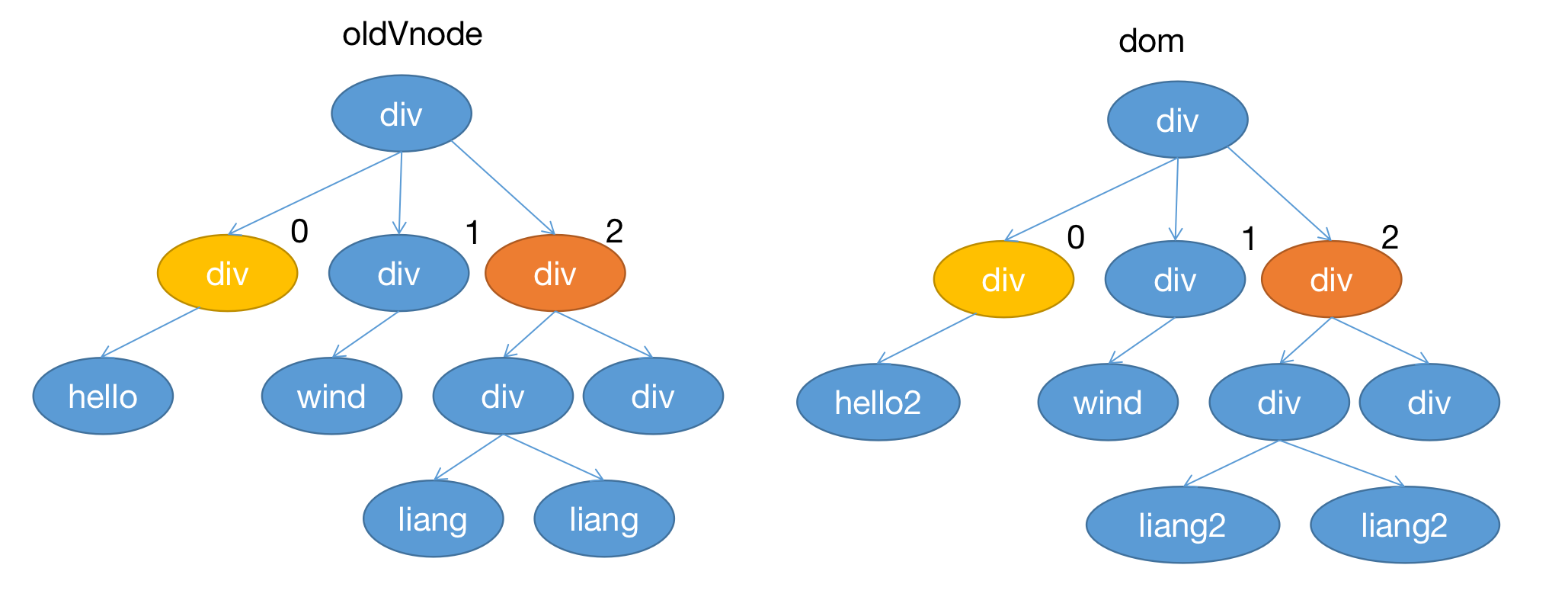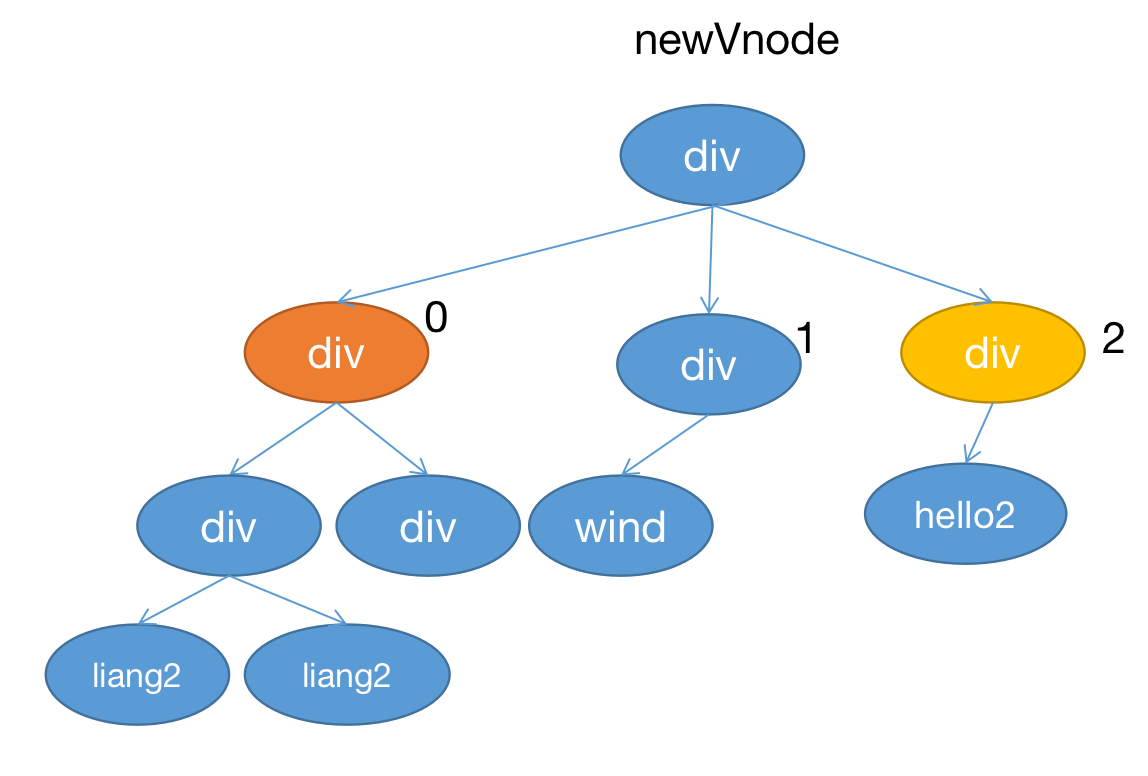## # diff 算法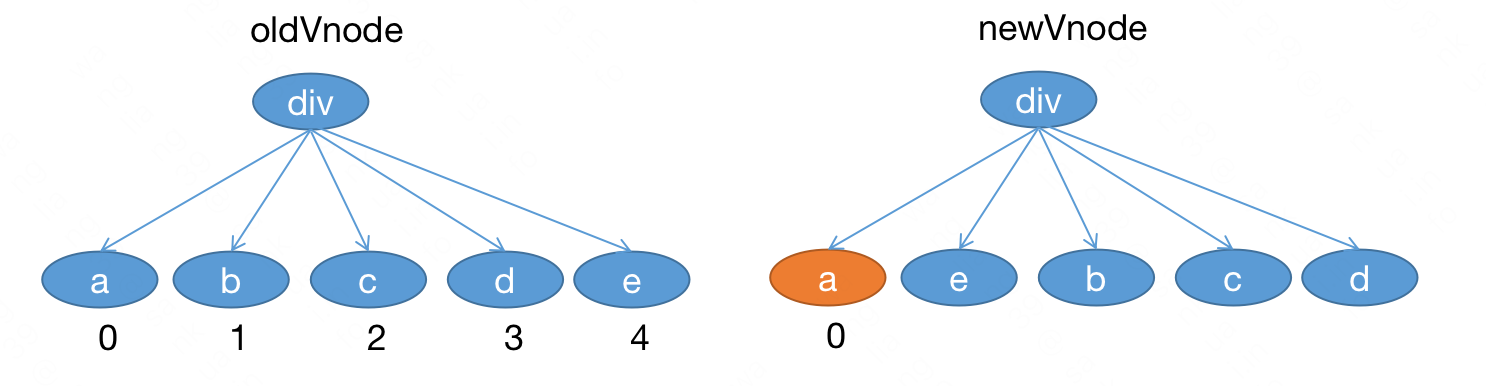### # 遍历到 e :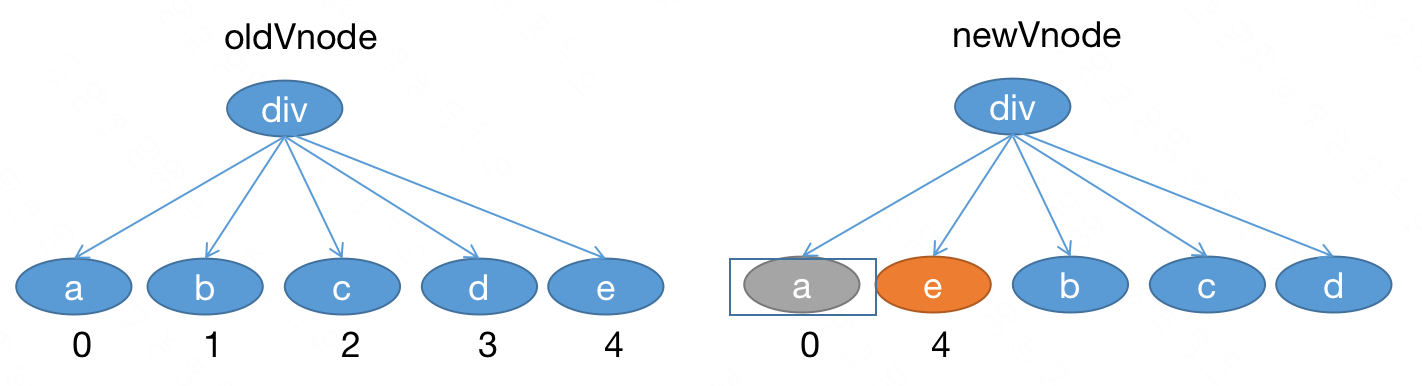newVnodeea 的后边， a 对应原来的位置是 0e 对应原来的位置是 4 ，说明原来 e 也在 a 后边，此时无需移动。

### # 遍历到 b: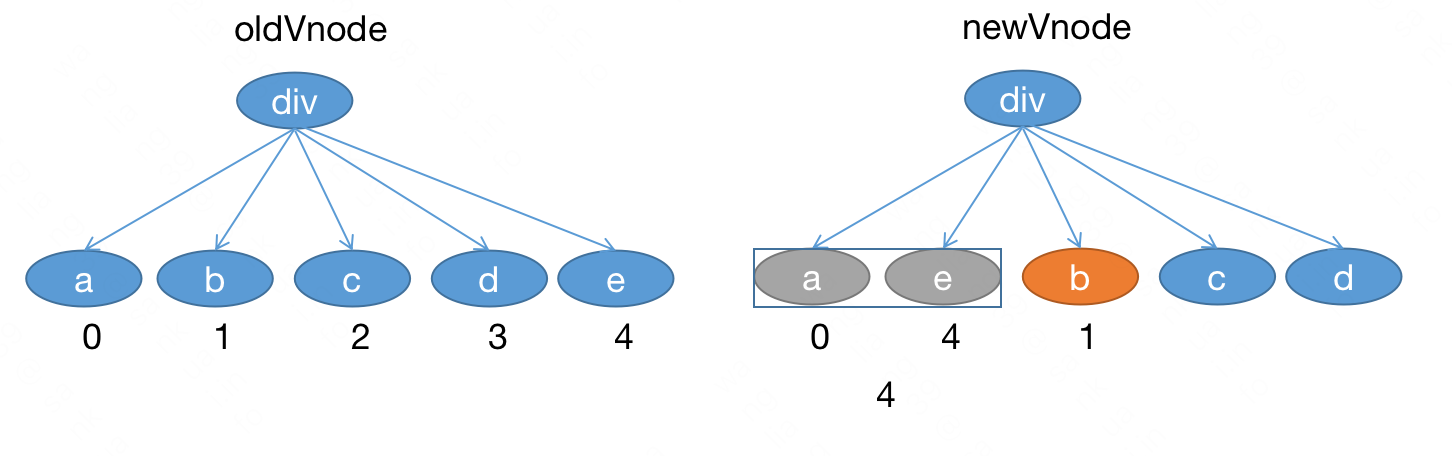newVnodeb 此时在 ae 的后边，如果 b 不用移动，那么 b 对应的原来的位置应该比 ae 对应的原来的位置都要大。

a 对应的原来位置是 0e 对应的原来的位置是 4 ，较大的位置是 4b 对应的原来的位置是 1 小于 4，说明 b 之前在 a 或者 e 的前边。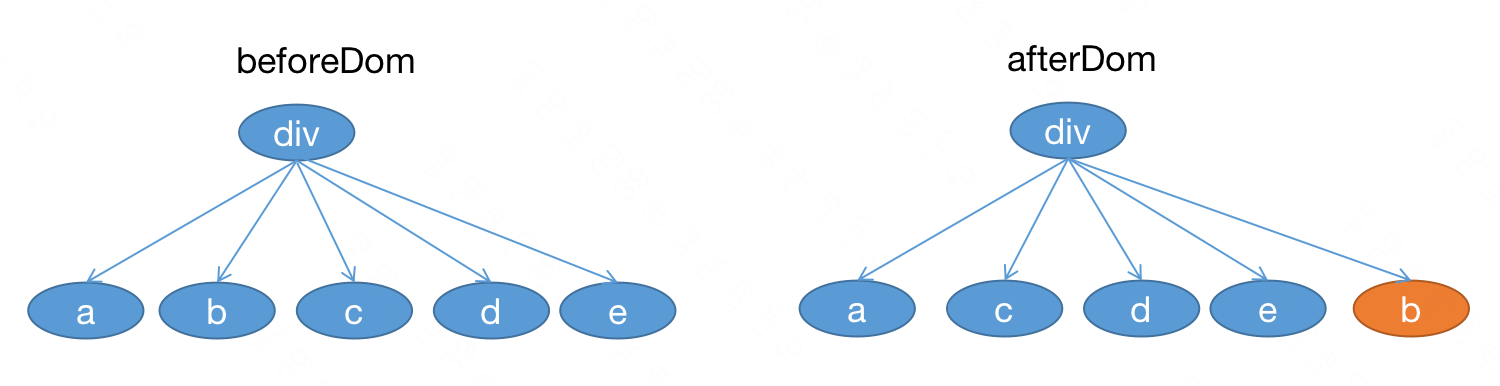### # 遍历到 c ：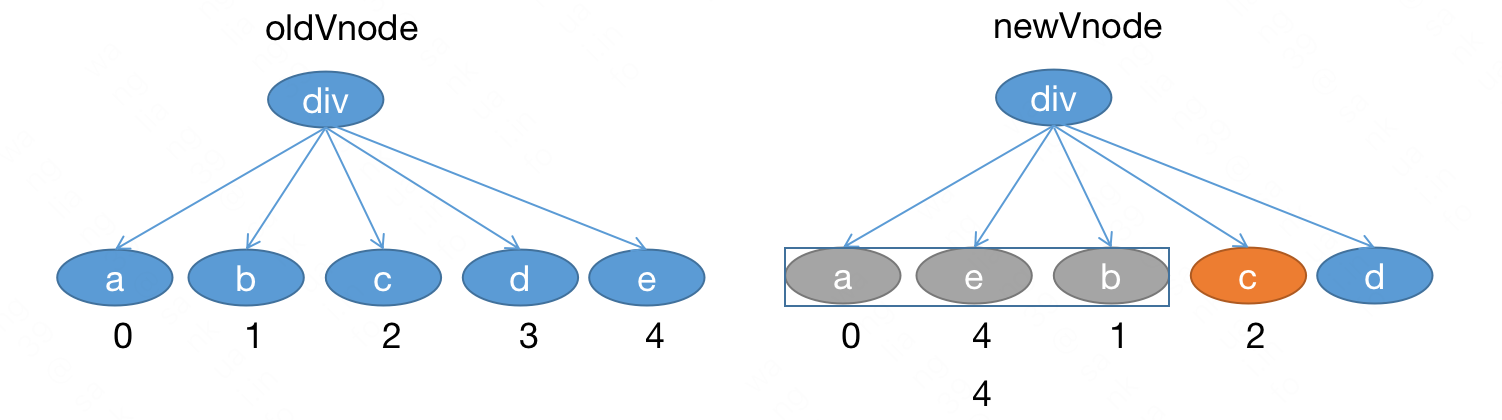c 此时在 aeb 的后边，如果 c 不用移动，那么 c 对应的原来的位置应该比 aeb 对应的原来的位置都要大。

a 对应的原来位置是 0e 对应的原来的位置是 4b 对应的原来的位置是 1，最大的位置是 4c 对应的原来的位置是 2 小于 4，说明 c 之前在 a 或者 e 或者 b 的前边。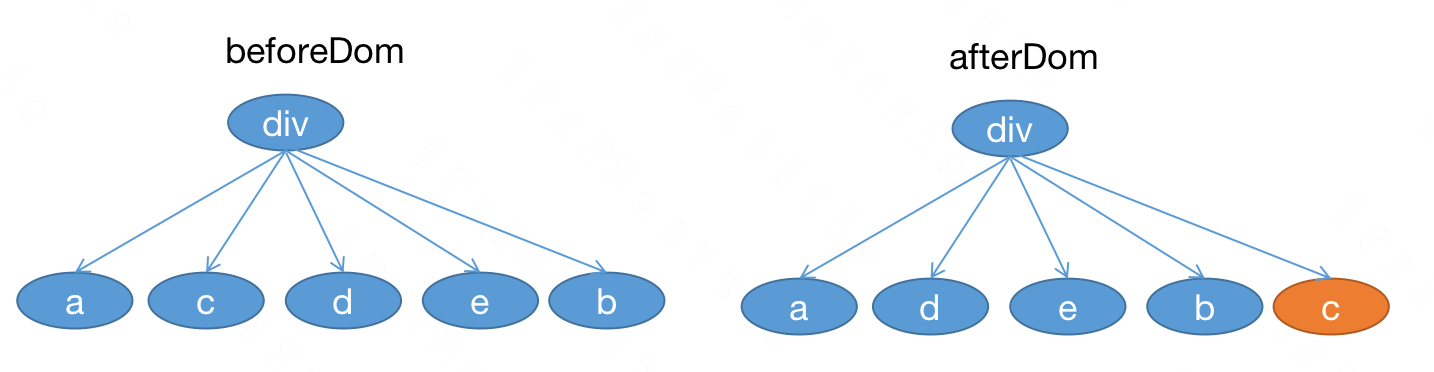### # 遍历到 d :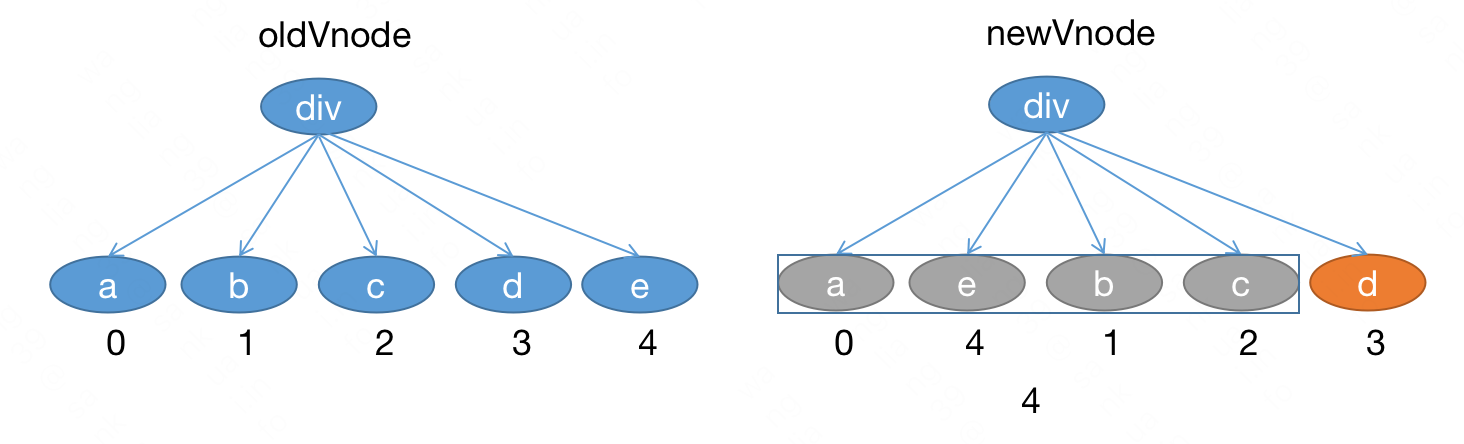d 此时在 aebc 的后边，如果 d 不用移动，那么 d 对应的原来的位置应该比 aebc 对应的原来的位置都要大。

a 对应的原来位置是 0e 对应的原来的位置是 4b 对应的原来的位置是 1c 对应的原来的位置是 2，最大的位置是 4d 对应的原来的位置是 3 < 最大位置 4 ，说明 d 之前在 a 或者 e 或者 b 或者 c 的前边。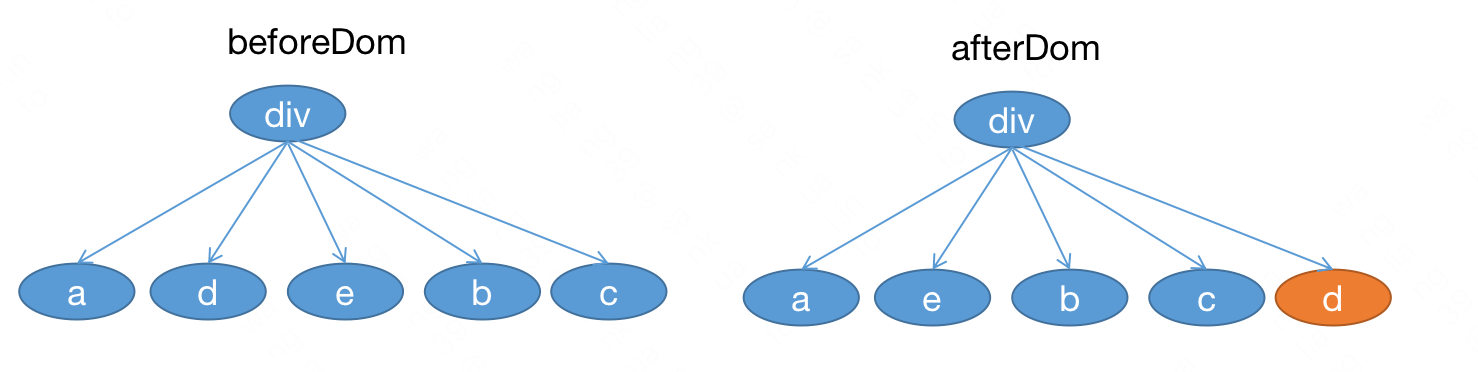### # 算法总结

function updateChildren(elm, oldCh, ch) {
let beforeMaxIndex = -1;
for (let i = 0; i < ch.length; i++) {
for (let j = 0; j < oldCh.length; j++) {
if (sameVnode(ch[i], oldCh[j])) {
ch[i].elm = oldCh[j].elm;
patchVnode(oldCh[j], ch[i]);
// 移动位置
if (i > beforeMaxIndex) {
// 无需移动
beforeMaxIndex = j;
} else {
const currentVnode = ch[i];
const beforeVnode = ch[i - 1];
nodeOps.insertBefore(
elm,
currentVnode.elm,
nodeOps.nextSibling(beforeVnode.elm)
);
}
break;
}
}
}
}


function createKeyToOldIdx(children, beginIdx, endIdx) {
let i, key;
const map = {};
for (i = beginIdx; i <= endIdx; ++i) {
key = children[i].key;
if (isDef(key)) map[key] = i;
}
return map;
}
// 没有 key 的话还是 for 循环
function findIdxInOld(node, oldCh, start, end) {
for (let i = start; i < end; i++) {
const c = oldCh[i];
if (isDef(c) && sameVnode(node, c)) return i;
}
}
function updateChildren(elm, oldCh, ch) {
let beforeMaxIndex = -1;
let oldKeyToIdx, idxInOld;
for (let i = 0; i < ch.length; i++) {
const newVnode = ch[i];
if (isUndef(oldKeyToIdx)) // 是否已经映射过了
oldKeyToIdx = createKeyToOldIdx(oldCh, 0, oldCh.length - 1);
idxInOld = isDef(newVnode.key)
? oldKeyToIdx[newVnode.key] // 有 key 直接映射
: findIdxInOld(newVnode, oldCh, 0, oldCh.length); // 没有 key 的情况

if (sameVnode(newVnode, oldCh[idxInOld])) {
newVnode.elm = oldCh[idxInOld].elm;
patchVnode(oldCh[idxInOld], ch[i]);
// 移动位置
if (i > beforeMaxIndex) {
// 无需移动
beforeMaxIndex = idxInOld;
} else {
const currentVnode = newVnode;
const beforeVnode = ch[i - 1];
nodeOps.insertBefore(
elm,
currentVnode.elm,
nodeOps.nextSibling(beforeVnode.elm)
);
}
}
}


## # 测试

import * as nodeOps from "./node-ops";
import modules from "./modules";
import { createPatchFunction } from "./patch";
import { createElement } from "./create-element";
import { observe } from "./observer/reactive";
import Watcher from "./observer/watcher";
const options = {
el: "#root",
data: {
list: ["a", "b", "c"],
},
render(createElement) {
const children = [];
for (const item of this.list) {
children.push(createElement("div", { key: item }, item));
}
const vnode = createElement(
"div",
{
on: {
click: () => {
console.log(1);
this.list = ["c", "b", "a"];
},
},
},
children
);
return vnode;
},
};

const _render = function () {
const vnode = options.render.call(options.data, createElement);
return vnode;
};

const __patch__ = createPatchFunction({ nodeOps, modules });

const vm = {};
vm.$el = document.querySelector(options.el); const _update = (vnode) => { const prevVnode = vm._vnode; vm._vnode = vnode; // Vue.prototype.__patch__ is injected in entry points // based on the rendering backend used. if (!prevVnode) { // initial render vm.$el = __patch__(vm.$el, vnode); } else { // updates vm.$el = __patch__(prevVnode, vnode);
}
};

observe(options.data);

new Watcher(options.data, () => _update(_render()));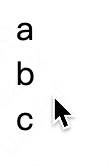dom 顺序成功进行了更新。

## # 总

Vue2 中对上边的 diff 算法进行了进一步的优化，下篇文章继续。

Last Updated: 6/14/2022, 9:37:44 AM